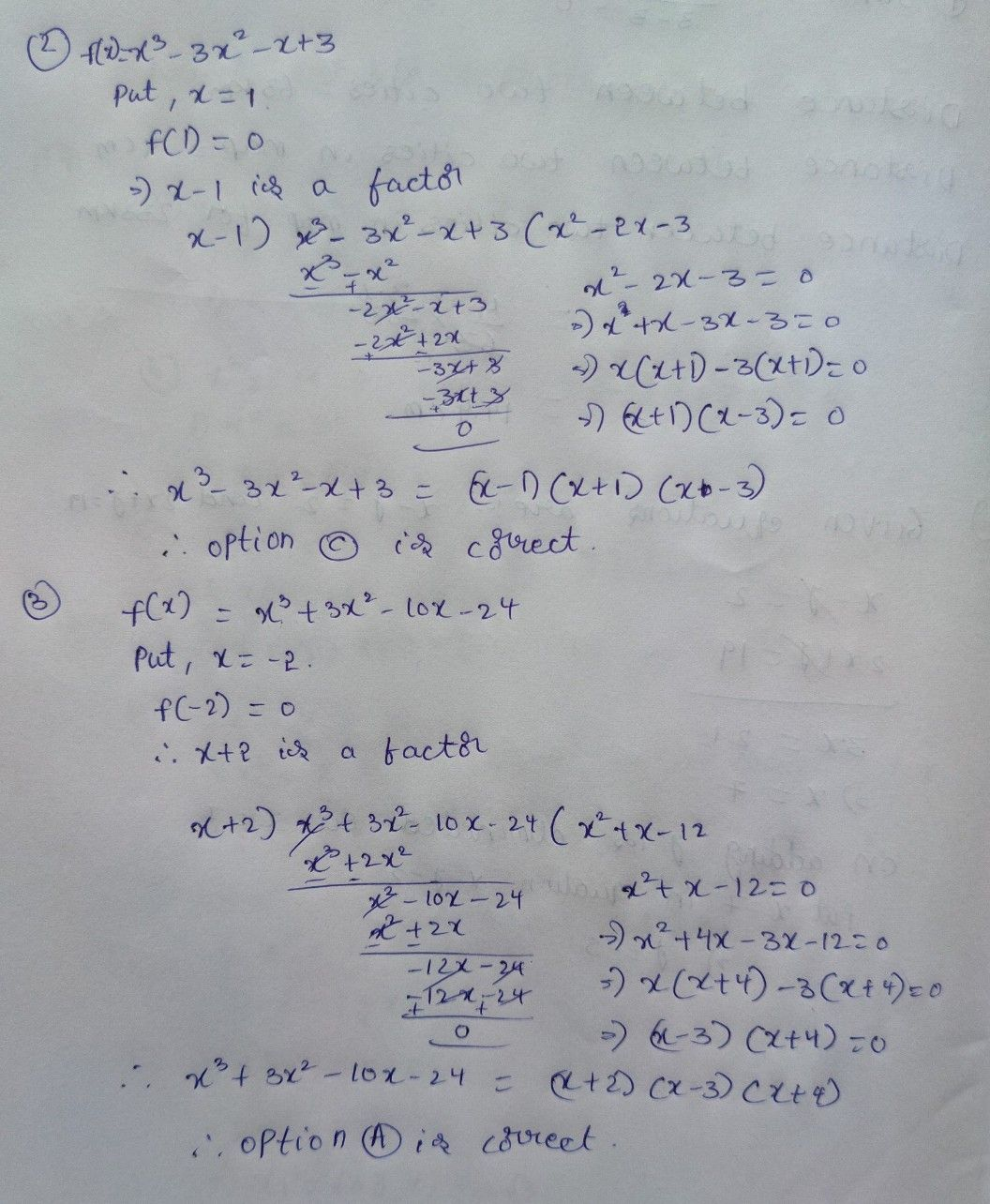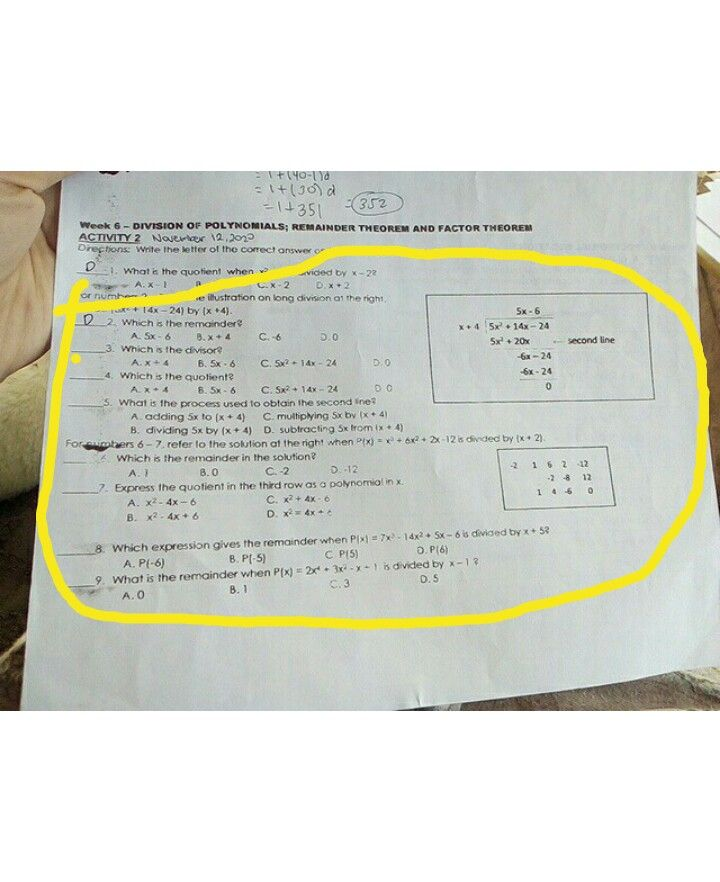Symbol
ProblemTaal atge 10. Which of the following is not a tactor of $4-x2.1\right)x2-9x$ D.x A.X-3 $1x2-9x+182$ B. x+3 C x-2 -2 ek 7- FACTORING POLYNOMIALS TIVITY 3 $30xx$ $s$ $1A.2020$ ctions: Write the letter of the correct answer on the biank before each number Show your solution on the oints each) 1. What are $1n0ioctooo12x^{2+7x+62}$ A. $\left(2x+3\right)\left(x+2\right)$ $8.\left(2+3\right)\left(x-2\right)$ $C\left(2x-3\right)\left(x-2\right)$ $0.\left(2x-3\right)b.2\right)$ 18 $2$ $Focto$ $x3-3x2-x+3$ $A.\left(x+3\right)\left(x-1\right)\left(x-1\right)$ $6.\left(x+3\right)\left(x-1\right)\left(x+1$ $C$ $\left(x-3\right)\left(x-1\right)\left(x+1\right)$ D. $\left(x-3\right)\left(x-1\right)\left(x-1\right)$ of x' 3. Which of the following is the factored form A. (x+ $4\right)\left(x-3\right)\left(x+2\right)$ (Sto CO (.(xx+ - 44) ) $3x2-10-242$ $\left(x-3\right)\left(x\dfrac {x} {x}21$ $x+31\left(-2\right)$ (x +2) B. (x - 4) (x-3) POLYNOMIAL EQUATIONS Y4 Norber 21.2020 ons: Complete the table below by identitying the degree and real roots of polynomial equations. write multiplicity 2, if it occurs thrice, write multiplicitv 3, and so on. Two examples are given to guide Polynomial Equation Degree Real Roots $x\left(5x-2\right)\left(x+8\right)=0$ 0.28 $\left(x-1\right)^{4}=0$ $\dfrac {\left(x+6\right)^{3}} {1\left(x+5\right)\left(x-7\right)\left(x+4\right)=0}$ 7. -6 multiolicity 3, 1 multiplicity 4 2. 3. $\dfrac {\left(x-11\right)\left(x-6\right)^{2}=0} {\left(x+9\right)^{5}=0}$
10th-13th grade
Other
Search count: 119
SolutionQanda teacher - dvivek231Studentmay I ask sir, what are the possible answer on the sec. pic.